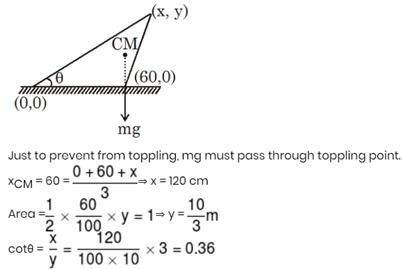Courses

# JEE Main Physics Mock - 8

## 25 Questions MCQ Test Mock Test Series for JEE Main & Advanced 2022 | JEE Main Physics Mock - 8

Description
This mock test of JEE Main Physics Mock - 8 for JEE helps you for every JEE entrance exam. This contains 25 Multiple Choice Questions for JEE JEE Main Physics Mock - 8 (mcq) to study with solutions a complete question bank. The solved questions answers in this JEE Main Physics Mock - 8 quiz give you a good mix of easy questions and tough questions. JEE students definitely take this JEE Main Physics Mock - 8 exercise for a better result in the exam. You can find other JEE Main Physics Mock - 8 extra questions, long questions & short questions for JEE on EduRev as well by searching above.
QUESTION: 1

Solution:
QUESTION: 2

### The working of dynamo is based on principle of

Solution: The dynamo operates on the principle of the production of dynamically induced emf. Hence when ever flux is cut by the conductor, emf is poduced in it according to the law of electromagnetic induction.
QUESTION: 3

### A constant force acts on a body of mass 0.9 kg at rest for 10 s. If the body moves a distance of 250 m, the magnitude of the force is

Solution:
Here d= 250m
t= 10 seconds
initial velocity(u)= 0
using equation d= ut +1/2 at2, we will get
250 = 50 xa
a=5m/s2
force= mass x acceleration = 0.9ks x 5m/s2 = 4.5 newton
QUESTION: 4

Two bodies of mass 10 kg and 5 kg moving in concentric orbits of radii R and r such that their periods are the same. Then the ratio between their centripetal acceleration is

Solution: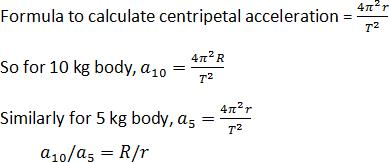QUESTION: 5
The directions of electric and magnetic fields in J.J. Thomson's experiment for the determination of e/m for an electron are
Solution: The specific charge of an electron can be determined when electron moves in both magnetic field and electric field which are mutually perpendicular to each other so that the net force on the electron is made zero. IN this situation the direction of motion of electron remains perpendicular to both electric and magnetic field.
QUESTION: 6

An electric dipole is placed along the X-axis at the origin O. A point P is at a distance of 20 cm from this origin such that OP makes an angle π/3 with the X axis. If electric field at P makes an angle θ with X-axis, the value of θ is

Solution: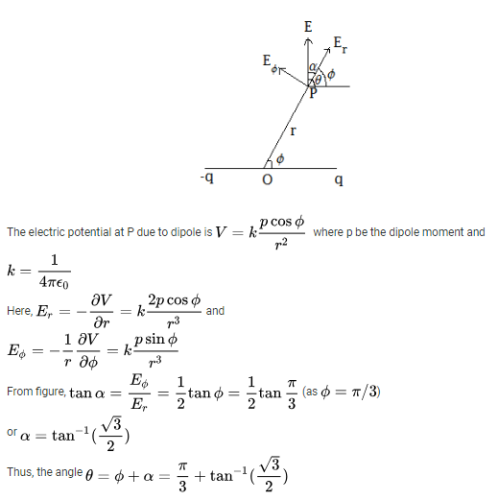QUESTION: 7
A glof ball of mass 0.05 kg placed on a tee, is struck by a golf club. The speed of the golf ball as it leaves the tee is 100 m/s, the time of contact between them is 0.02 s. If the force decreases to zero linearly with time, then the force at the beginning of the contact is
Solution:
QUESTION: 8

A semi circle arc of radius 'a' is charged uniformly and the charge per unit length is λ. The electric field at its centre is

Solution:
QUESTION: 9

The figure shows the symbol of a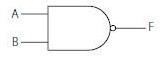Solution:
QUESTION: 10
A liquid flows through a pipe of non-uniform cross-section. If A₁ and A₂ are the cross-sectional areas of the pipe at two points, the ratio of velocities of the liquid at these points will be
Solution:
QUESTION: 11
A particle is moving in a uniform magnetic field then
Solution:
QUESTION: 12

Which of the following equations is definitely wrong?

Solution:
QUESTION: 13
In neutron discovery experiment, Berillium element is bombarded by
Solution:
QUESTION: 14
In the following question, a Statement of Assertion (A) is given followed by a corresponding Reason (R) just below it. Read the Statements carefully and mark the correct answer-
Assertion(A): A particle move from A (X1,Y1,Z1) to B (X2,Y2,Z2), displacement is given as (X2-X1)i, (Y2-Y1)j, (Z2-Z1)k.
Reason (R):Displacement can be positive, negative or zero.
Solution:
QUESTION: 15
In the following question, a Statement of Assertion (A) is given followed by a corresponding Reason (R) just below it. Read the Statements carefully and mark the correct answer-
Assertion(A): The density of air at the top of the troposphere is about 10 times the density near the earth's surface.
Reason(R): The atmosphere between the heights of 12 km and 50 km is called troposphere.
Solution:
QUESTION: 16

For a gas, if the ratio of specific heats at constant pressure and volume is γ, then the value of degree of freedom is

Solution:

Cp=(f/2+1)RT
Cv= (f/2)RT
Taking ratio we get
Cp/Cv = y = (f/2 + 1)/(f/2)
y=(f + 2)/f
y=1 + 2/f
y -1=2/f
f = 2/(y-1)
Hope it helps

QUESTION: 17

A 2 kg mass is rotating on a circular path of radius 0.8 m with angular velocity of 44 rad-s-1. If radius of the path becomes 1 m, then value of angular velocity will be

Solution: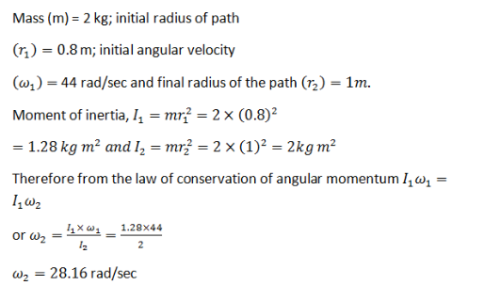QUESTION: 18

Which is not a unit of electric field

Solution:
QUESTION: 19
Ultrasonic waves are those waves
Solution:
QUESTION: 20

In a mixture of H−He+ gas (He+ is singly ionized He atom) , H atoms and He+ ions are excited to their respective first excited states. Subesquently, H atoms transfer their total excitation energy to He+ ions (by collisions). Assume that the Bohr model of atom is exactly vaild.

Q. The quantum number n of the state finally populated in He+ ions is

Solution:
*Answer can only contain numeric values
QUESTION: 21

A biconvex thin lens is prepared from glass of refractive index µ2 = 3/2. The two converging surface have equal radii of  20cm each. One of the surface is silvered from outside to make it reflecting. It is placed in a medium of refractive index µ1 = 5/3. This system will behave as concave mirror of focal length f, find value of |f| in cm.

Solution: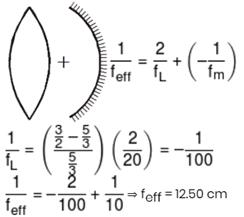*Answer can only contain numeric values
QUESTION: 22

Find the maximum kinetic energy (in eV) of the photoelectron liberated from the surface of lithium (work function φ = 2.15eV) by electromagnetic radiation whose electric component varies with time as E = a (1 + cos ωt)cosω0t,
where a is a constant, ω = 12 × 1014 rads–1 and ω0 =  3.6 × 1015 rads–1 (h = 6.6 × 10–34 in SI units)

Solution: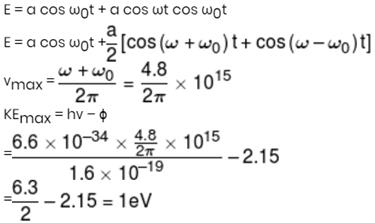*Answer can only contain numeric values
QUESTION: 23

A photosensitive surface is irradiated with light of wavelength λ, the stopping potential is V. When the same surface is irradiated with the light of wavelength 2λ, stopping potential is V/3. Then the ratio of threshold wavelength (λmax) and the λ is

Solution: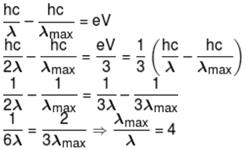*Answer can only contain numeric values
QUESTION: 24

One of the circuits for the measurement of resistance by potentiometer is shown. The galvanometer is connected at point A and zero deflection is observed at length PJ = 30 cm. In second case the secondary cell is changed. Take ES = 10 V and r = 1Ω  in 1st reading and ES = 5V and r = 2Ω in 2nd reading. In second case, the zero deflection is observed at length PJ = 10 cm. What is the resistance R (in ohm)?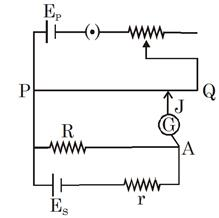Solution: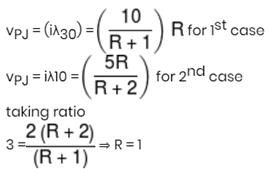*Answer can only contain numeric values
QUESTION: 25

A uniform triangular plate of triangular area 1m2, base length 60 cm and thickness 10 mm (prism like shape) is lying vertically on a smooth ground as shown in figure. Find maximum value of cotθ for which it does not topple.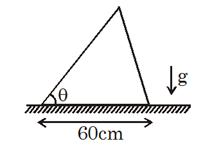Solution: